# 0 1 Let S span 1 1 1 0 }, a basis for S. Show that| (a) Let B1 { 1 0 1 1 0 is also a basis for S 0 B2 { 1 (b) Write eac...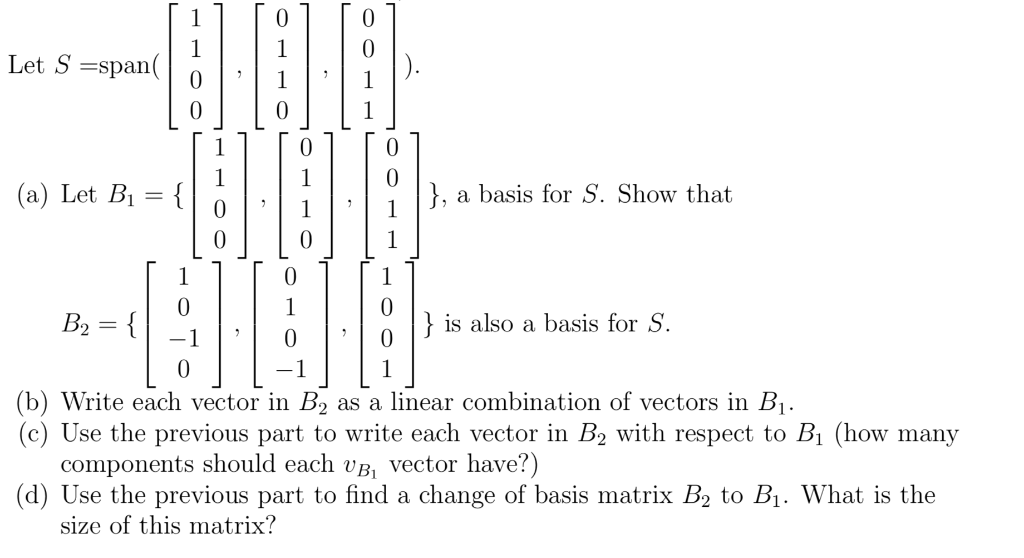0 1 Let S span 1 1 1 0 }, a basis for S. Show that| (a) Let B1 { 1 0 1 1 0 is also a basis for S 0 B2 { 1 (b) Write each vector in B2 (c) Use the previous part to write each vector in B2 with respect to Bi (how many components should each vB, vector have?) (d) Use the previous part to find a change of basis matrix B2 to B1. What is the as a linear combination of vectors in B1. size of this matrix?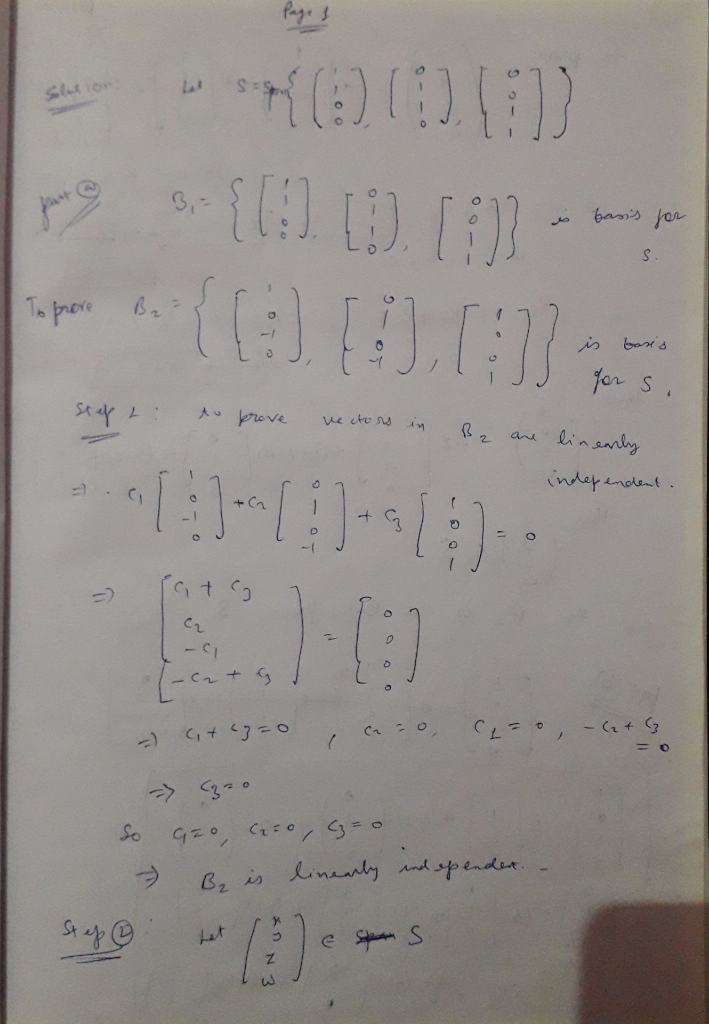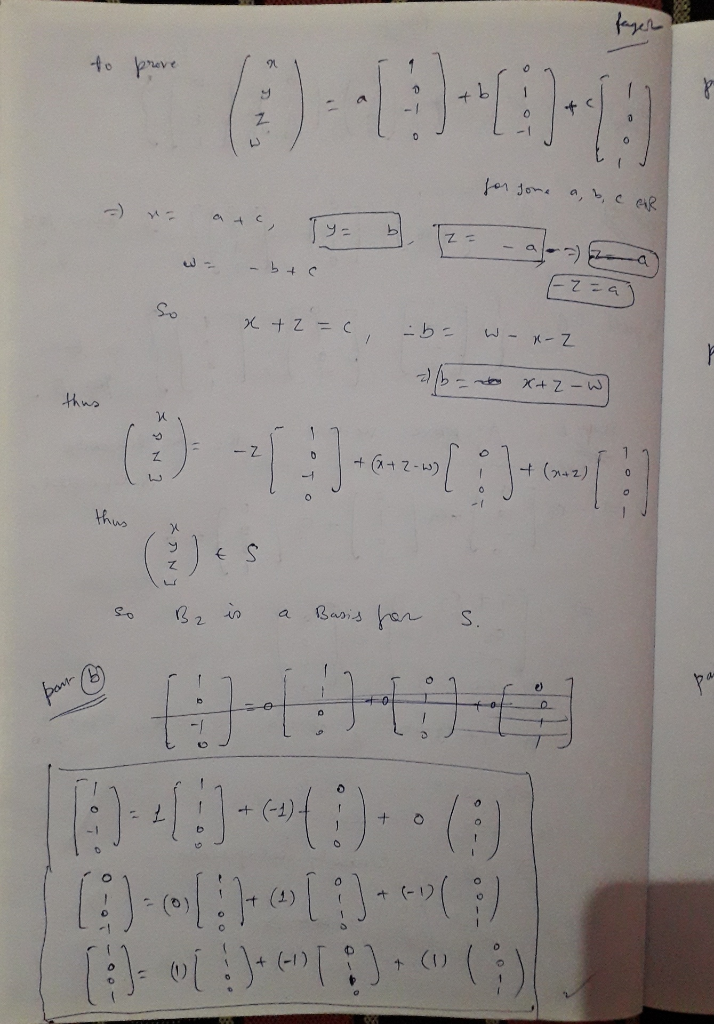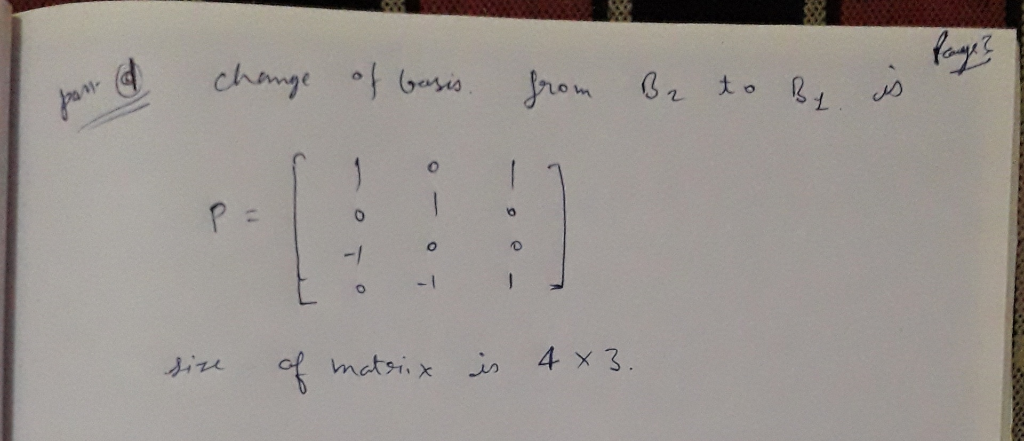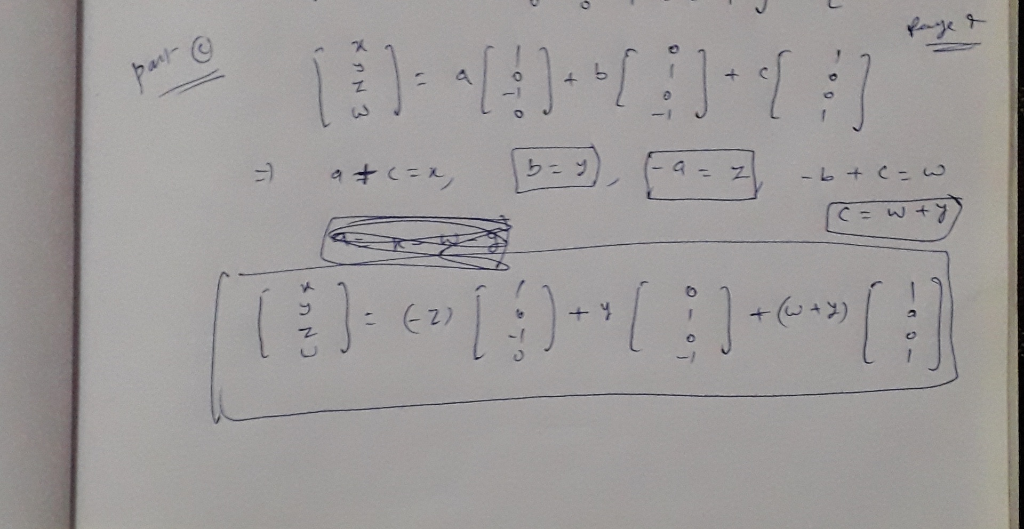#### Earn Coin

Coins can be redeemed for fabulous gifts.

Similar Homework Help Questions
• ### Let B = {b1,b2, b3} be a basis for a vector space V. Let T be...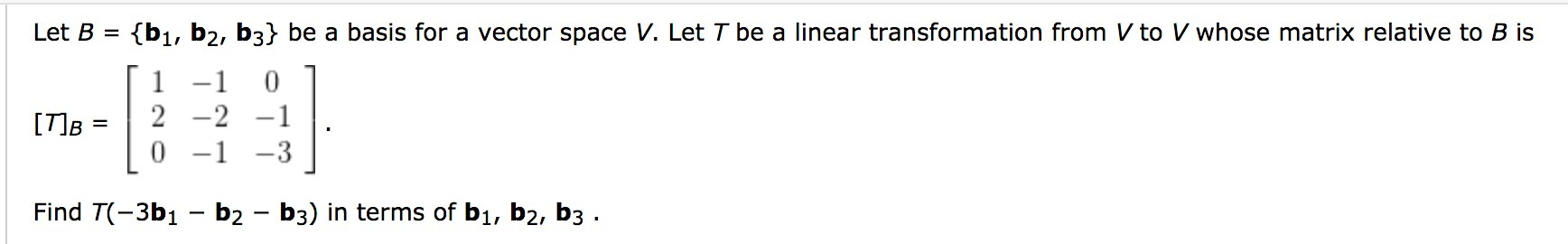Let B = {b1,b2, b3} be a basis for a vector space V. Let T be a linear transformation from V to V whose matrix relative to B is [ 1 -1 0 1 [T]B = 2 -2 -1 . 10 -1 -3 1 Find T(-3b1 – b2 - b3) in terms of bı, b2, b3 .

• ### (b) Let E = {(1, C2, C3} be the standard basis for R3, B = {bų,...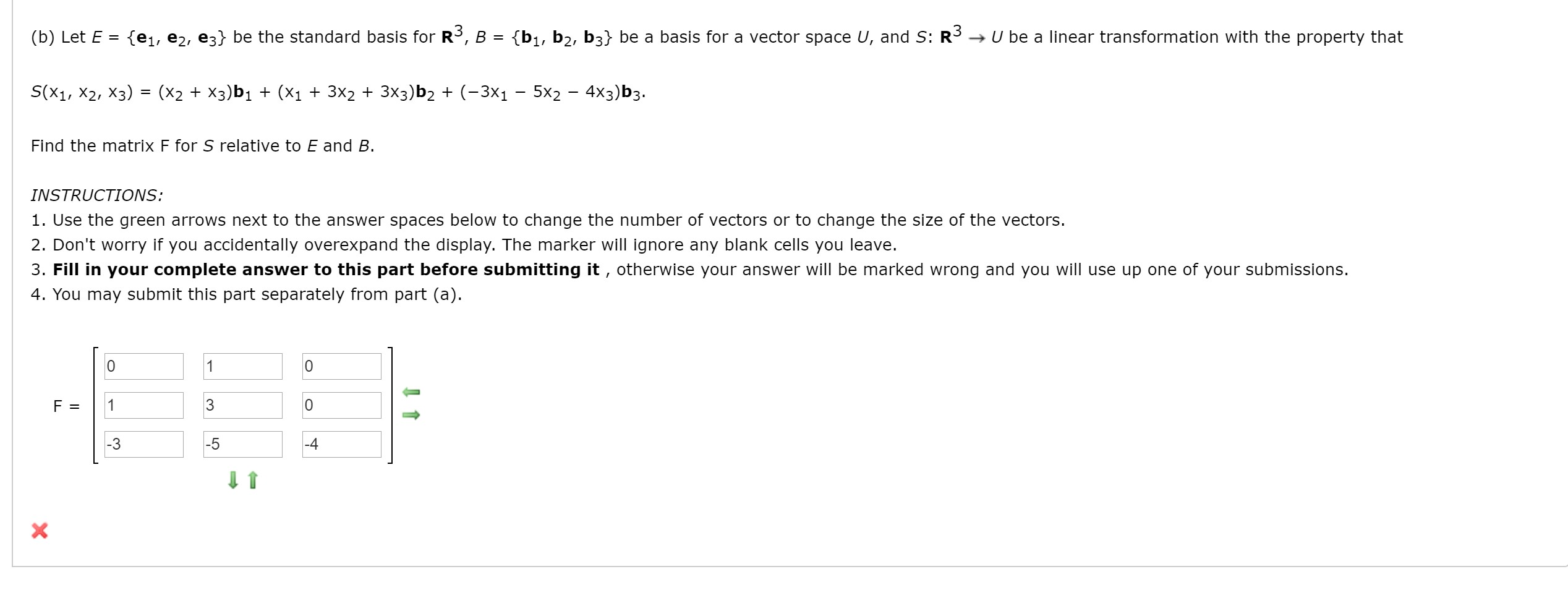(b) Let E = {(1, C2, C3} be the standard basis for R3, B = {bų, b2, b3} be a basis for a vector space U, and S: R3 → U be a linear transformation with the property that S(X1, X2, X3) (x2 + x3)b1 + (x1 + 3x2 + 3x3)b2 + (-3X1 - 5x2 - 4x3)b3. Find the matrix F for S relative to E and B. INSTRUCTIONS: 1. Use the green arrows next to the answer spaces below...

• ### How was the linear transformation of b1 and b2 were applied (L(b1) , L(b2))? NOTE: b1=(1,1)^T , b2=(-1,1)^T Linear Transformations EXAMPLE 4 Let L be a linear transformation mapping R? into itself an...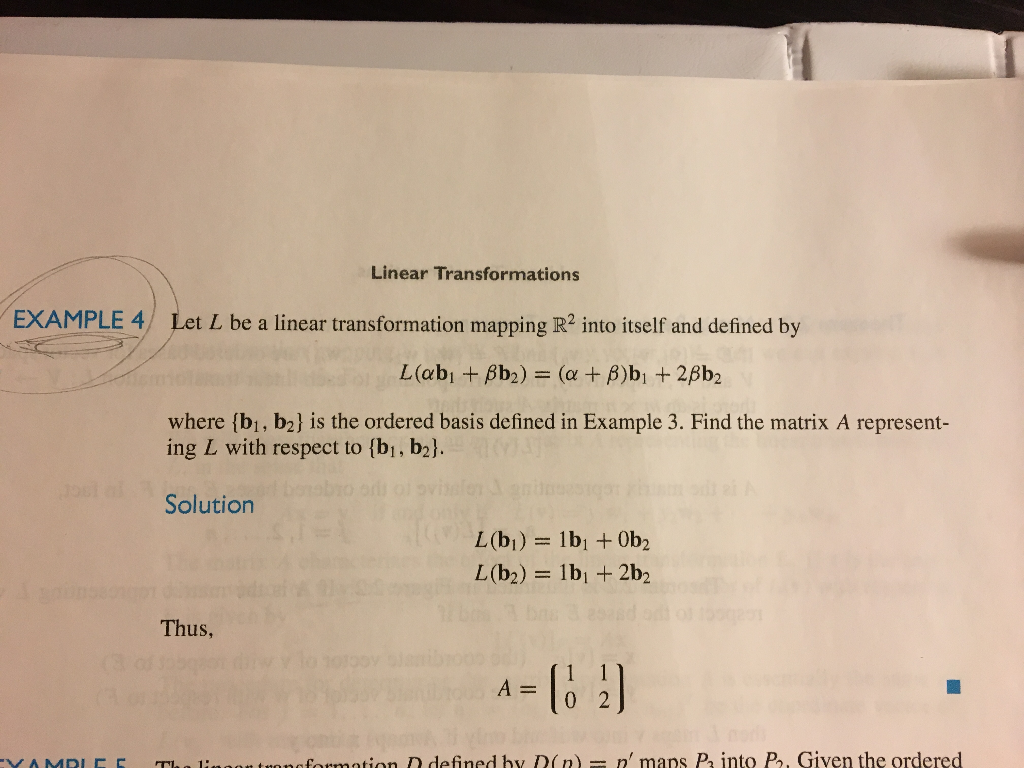How was the linear transformation of b1 and b2 were applied (L(b1) , L(b2))? NOTE: b1=(1,1)^T , b2=(-1,1)^T Linear Transformations EXAMPLE 4 Let L be a linear transformation mapping R? into itself and defined by where (bi, b2] is the ordered basis defined in Example 3. Find the matrix A represent- ing L with respect to [bi, b2l Solution Thus, A0 2 onofosmation D defined by D(n n' maps P into P, Given the ordered Linear Transformations EXAMPLE 4 Let...

• ### linear algebra do all parts A,B,C and D please 1. Let B = {bi, b2)- and...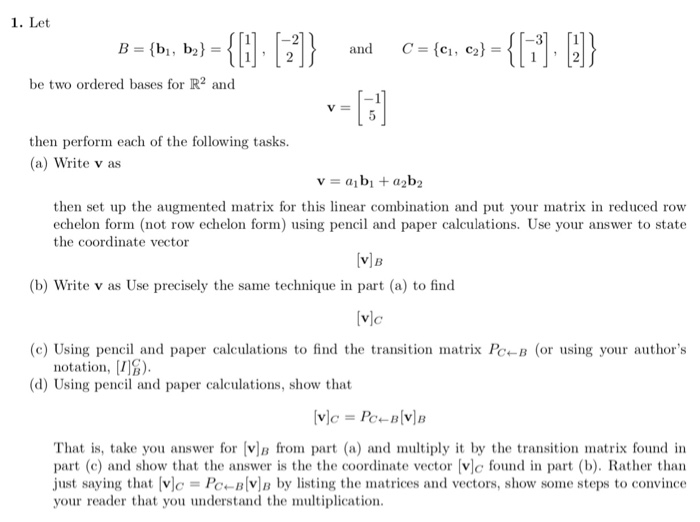linear algebra do all parts A,B,C and D please 1. Let B = {bi, b2)- and C-(C1 , С2)- 111,12 be two ordered bases for R2 and VE then perform each of the following tasks. (a) Write v as then set up the augmented matrix for this linear combination and put your matrix in reduced row echelon form (not row echelon form) using pencil and paper calculations. Use your answer to state the coordinate vector VB (b) Write v as...

• ### Assume that the transition matrix from basis B = {b1, b2, b3} to basis C = {c1, c2, c3} is PC,B = 1/2*[ 0 -1 1 ; -1 1 1 ; 1 0 0 ]. (a) If u = b1 + b2 + 2b3, find [u]C. (b) Calculate PB,C. (c) Suppose...

Assume that the transition matrix from basis B = {b1, b2, b3} to basis C = {c1, c2, c3} is PC,B = 1/2*[ 0 -1 1 ; -1 1 1 ; 1 0 0 ]. (a) If u = b1 + b2 + 2b3, find [u]C. (b) Calculate PB,C. (c) Suppose that c1 = (1, 2, 3), c2 = (1, 2, 0), c3 = (1, 0, 0) and let S be the standard basis for R 3 . (i) Find...

• ### 1) for R2 Given the vectors b1,b2, C1, and cz. B = {b1,b2} is a basis...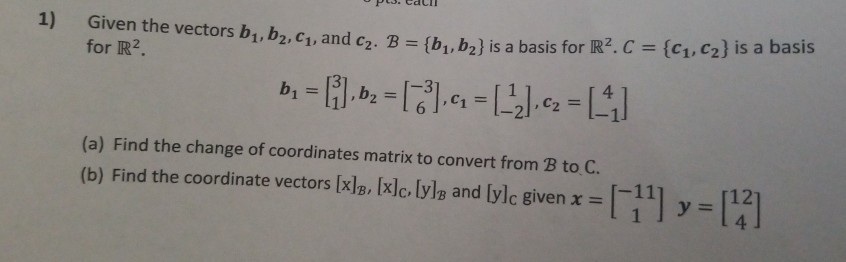1) for R2 Given the vectors b1,b2, C1, and cz. B = {b1,b2} is a basis for R2C = {C1,C2} is a basis b = [i.bz = ,4 = (-2) c2 =  (a) Find the change of coordinates matrix to convert from B to C. (b) Find the coordinate vectors [x]B, [x]c, lyle and [ylc given x =  y = 

• ### solution of question d (4 points) Consider the basis of R5 given by with b2 (2,-1,-5,-4,7), b3-(3, 2,-7,-5,9) b4 2,1,4,...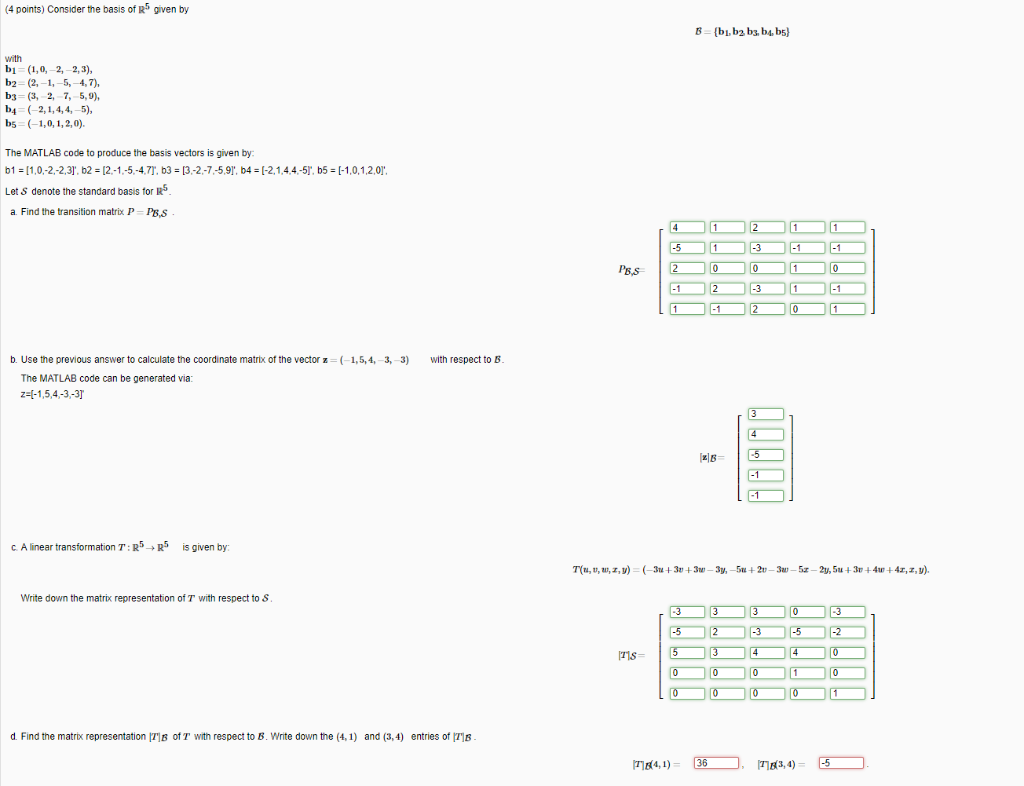solution of question d (4 points) Consider the basis of R5 given by with b2 (2,-1,-5,-4,7), b3-(3, 2,-7,-5,9) b4 2,1,4,4,-5) bs (-1,0,1,2,0) The MATLAB code to produce the basis vectors is given by b1 11,0-2-2.3], b2 -12-1.-5-4,7T, b3 13-2-7-5,91, b4 [-2,14.4-5T, b5 1-1,0,1,20 Let S denote the standard basis for R a Find the transition matrix P P,s PB,s b. Use the previous answer to calculate the coordinate matrix of the vector z ( 1,5, 4, 3, 3) with respect...

• ### 1. Given the spring-mass-damper system in the figure below T3 T1 T2 b2 b1 k3 (a) Find the equatio...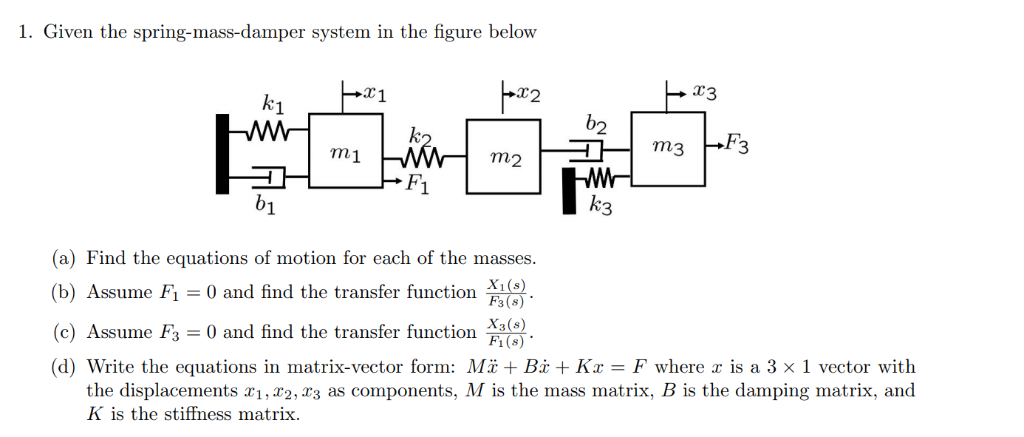1. Given the spring-mass-damper system in the figure below T3 T1 T2 b2 b1 k3 (a) Find the equations of motion for each of the masses 脳. Fi(s) (b) Assume F1 0 and find the transfer function (c) Assume Fs 0 and find the transfer function (d) Write the equations in matrix-vector form: M.ї + Bi + Kx-F where z is a 3 x 1 vector with the displacements r,2, r3 as components, M is the mass matrix, B is...

• ### Please show work Problem 2. Consider the vectors  1 1 v1 = 1, V2 =...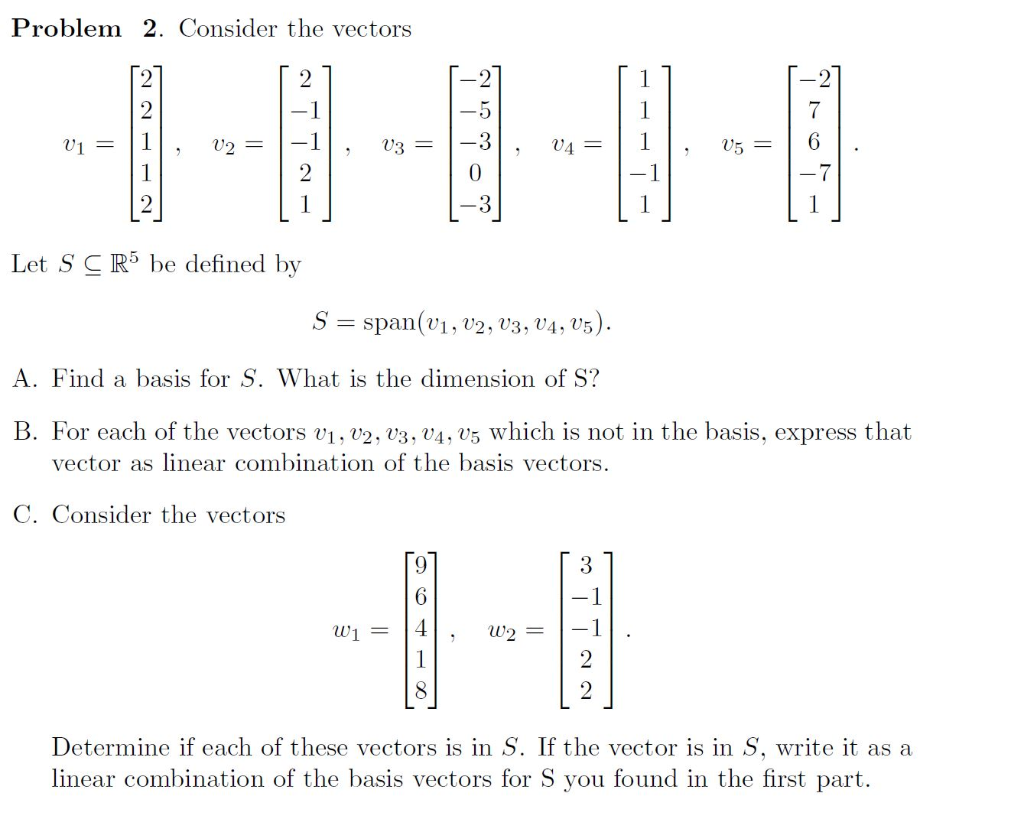Please show work Problem 2. Consider the vectors  1 1 v1 = 1, V2 = -1, V3 = -3 , 04 = , 05 = 6 Let S CR5 be defined by S = span(V1, V2, V3, V4, 05). A. Find a basis for S. What is the dimension of S? B. For each of the vectors V1, V2, V3, V4.05 which is not in the basis, express that vector as linear combination of the basis vectors. C. Consider...

• ### V1 = 1 , V2= -1 , U3 = , 04 = 1 , 05 =...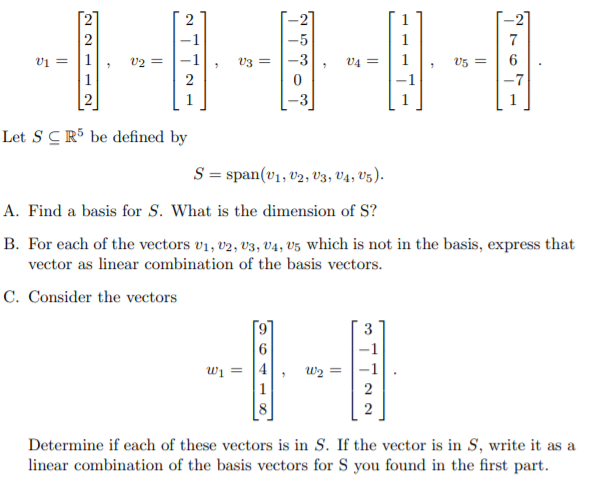V1 = 1 , V2= -1 , U3 = , 04 = 1 , 05 = 6 -3 0 | 2 Let S CR5 be defined by S = span(01, 02, 03, 04, 05). A. Find a basis for S. What is the dimension of S? B. For each of the vectors 01, 02, 03, 04, 05 which is not in the basis, express that vector as linear combination of the basis vectors. C. Consider the vectors W1 = 14,...